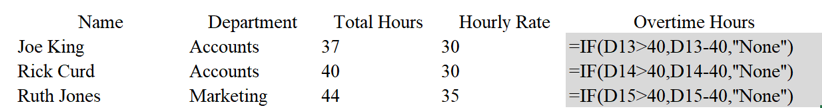# Excel IF Statements Tutorial

## Microsoft Excel Free Tips & Tutorials

Learn how to use IF statements in Microsoft Excel to return output based on the contents of another cell.

=IF(Logical Test,Value if True, Value if False)

Logical Test: Equality/Inequality statement on which the outcome is decided

Value if True: What action occurs of the logical test is true

Value if False: What action occurs of the logical test is falseOperator Meaning (Example)
"=" (equal to) Equal to (A1=B1)
>(greater than) Greater than (A1>B1)
< (less than) Less than (A1
>= (greater than or equal to) Greater than or equal to (A1>=B1)
<= (less than or equal to) Less than or equal to (A1<=B1)
<> (not equal to) Not equal to (A1<>B1)